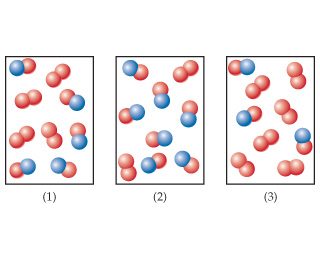# Problem: The following diagrams represent mixtures of NO(g) and O2(g). These two substances react as follows:2 NO ( g ) + O2 ( g ) → 2 NO2 ( g )It has been determined experimentally that the rate is second order in NO and first order in O2.Based on this fact, which of the following mixtures will have the fastest initial rate?

⚠️Our tutors found the solution shown to be helpful for the problem you're searching for. We don't have the exact solution yet.

###### Problem Details

The following diagrams represent mixtures of NO(g) and O2(g). These two substances react as follows:
2 NO ( g ) + O2 ( g ) → 2 NO2 ( g )
It has been determined experimentally that the rate is second order in NO and first order in O2.

Based on this fact, which of the following mixtures will have the fastest initial rate?